# How to draw a wiggle between two points with Python and Drawbot

## Sometimes a wiggle could be a good solution to emphasize a special connection between two points

In this tutorial we are going to use Python 2.7, Drawbot and some high-school geometry. The script is on GitHub, you can have it if you scroll down.

Any graphic designers reading will likely recall the wiggles trend from a few years ago. If not, take a look at this section on trendlist.org. Fashion trends aside, let’s say you need to emphasise the relationship between two points on a canvas, however, for whatever reason, color and thickness are not an option. You can only address the shape of the connection. I have never been a big fan of dashed lines — the canvas becomes fuzzy very quickly — so perhaps a wiggle line could be useful. Drawing this kind of path with a Bézier editor is very time consuming, so I’d like the computer to do the heavy lifting for us. This tutorial will cover my own implementation in Drawbot.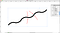Yes, InDesign supports wiggles. But these curves are just ugly. Granted, printed at 1pt with a laser digital printer these spikes will be all but invisible, but there’s room for improvement

First of all, it is important to determine which variables we wish to control. Obviously we will have a start and end point, as well as wave length and height. Why not wave count? Well, if I need to highlight multiple point pairings, wave density will be a more meaningful visual metric than wave count. I mean, who has time to count waves?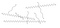Each wiggle has a slightly different wave length. Half of them belong to one group, the rest to another. Can you spot the difference?

We should not forget that when Bézier curves are used, we have to take care of bezier control points (referred to from here onwards as ‘bcp’).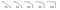My favourite way of dealing with this is by using the squaring approach: a ratio between 0 and 1 which defines the position of the bcp in relation to a maximum bcp length.

There is one caveat with this implementation. The distance between pt1 and pt2 divided by the wave length should not give a remainder. But that’s a big ask for real life use. Of course you can instruct the script to return an error (using the assert statement), but in most cases this would result in no line being drawn. In my opinion, the best thing to do is to round the wave length to an amount which allows an integer amount of waves in the wiggle.

Before diving into the code, I would like to spend a few extra words talking about bcp position. Twice each wave the segment that ideally connects pt1 and pt2 is divided with a point. This is the inflection point, whereby the Bézier curve changes direction. In order to calculate the position of the bcps projecting from the flex point we need to determine, in order: the inclination angle and the bcp length. With these values we can use sine and cosine to compute the coordinates.

The bcpInclination value is of course dependant on the angle of the wiggle line. Both angles are calculated using the atan2 function.

The maximum bcp length is instead computed using the Pythagorean theorem. It is the length of the hypotenuse of the right triangles in which is possible to divide the isosceles triangle where each Bézier curve is inscribed. The cathetus aligned with the wiggle is a quarter of the adjusted wave length, the other cathetus measures half of the wave height.

Once found the maximum bcp length we can compute the bcpLength as a simple multiplication. Now it is only a matter of iterating the calculation correctly across the wiggle.
So, let’s dive into the code, it’s time to open Drawbot.

In order to understand the following steps you should be familiar with the following Python topics: imports, for loops and functions. In case, Think Python by Allen B. Downey is a good resource to brush them up.

I usually organize my scripts into four sections: Modules, Constants, Functions/Procedures, Variables and Instructions. We need a number of functions from the Python math module. I also prefer storing points data into namedtuple instances from the collections module. It makes the code more readable when accessing the data inside.

`from math import radians, atan2, sqrt, sin, cosfrom collections import namedtuple`

Then we can define the Point namedtuple we are going to use and declare the two points which will be the extremes of the wiggle.

`Point = namedtuple('Point', ['x', 'y'])[…]pt1 = Point(50, 50)pt2 = Point(150, 60)`

In order to draw on the Drawbot canvas, we need to define a canvas size using the size() function. This function should always be the first call related to the Drawbot context, otherwise Drawbot will provide a standard canvas (1000x1000)

`size(400, 400)`

The drawing routine will be organized into two functions in order to make the code easily portable: one function (Drawbot independent) will calculate the points needed to draw the wiggle, and another function (Drawbot specific) will draw the points on the canvas.

`def calcWiggle(pt1, pt2, waveLength, waveHeight, curveSquaring=.57):    passdef drawCurveSequence(wigglePoints):    pass`

In order to define the context of the wiggle calculation, we must use the assert statement to detect possible mistakes. The squaring value should be between 0 and 1 and the wave length should be bigger than 0.

`assert 0 <= curveSquaring <= 1, 'curveSquaring should be a value between 0 and 1: {}'.format(curveSquaring)assert waveLength > 0, 'waveLength smaller or equal to zero: {}'.format(waveLength)`

The distance between pt1 and pt2 is needed in order to computer the adjusted wave length. I would rather keep this calculation in a separate function:

`def calcDistance(pt1, pt2):    return sqrt((pt1.x — pt2.x)**2 + (pt1.y — pt2.y)**2)`

Likewise for the angle calculation of the segment between two points:

`def calcAngle(pt1, pt2):    return atan2((pt2.y — pt1.y), (pt2.x — pt1.x))`

I use these functions everywhere in my code, means it’s possible to copy them in any Python script (don’t forget the imports). So we can now compute the distance between pt1 and pt2, and the inclination of the wiggle. Be aware, atan2 returns an angle value in radians.

`diagonal = calcDistance(pt1, pt2)angleRad = calcAngle(pt1, pt2)`

Before getting into the for loop which will iteratively compute the wiggle points, we need to define a few extra local variables. First the amount of waves — which will be used in order to define the for loop — and the adjusted wave interval. Please note the integer division // for the first instruction.

`howManyWaves = diagonal//int(waveLength)waveInterval = diagonal/float(howManyWaves)`

Consequently the ones needed for the bcps:

`maxBcpLength = sqrt((waveInterval/4.)**2+(waveHeight/2.)**2)bcpLength = maxBcpLength*curveSquaringbcpInclination = calcAngle(Point(0,0), Point(waveInterval/4., waveHeight/2.))`

Before launching the for loop, we need to initiate a list within which to store the Bézier curve (the only item already inside will be pt1 as moveTo() point), a variable name for the previous flex point (each flex point is located using the previous one), and a polarity variable which will switch between 1 and -1 in order to move the wiggle up and down. The minus signs in the following diagram are obtained by multiplying the resulting angle by the polarity variable. Take into account that the for loop has to iterate twice the wave amount, because we need two flex points for each wave.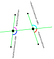The four angles needed to draw upwards and downwards
`wigglePoints = [pt1]prevFlexPt = pt1polarity = 1for waveIndex in range(0, int(howManyWaves*2)):    […]`

Each iteration will take care of computing the triplet of points needed to draw a Bézier curve: bcpOut (which is linked to the previous points in the postscript sequence), the bcpIn, and the end flex points. The triplets will be then stored as a tuple in the wigglePoints list.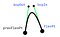The blue labels represent a Bézier triplet

Each pair of coordinates is defined using sine and cosine; take a look at this diagram if you need to brush up on your trigonometry.

`bcpOutAngle = angleRad+bcpInclination*polaritybcpOut = Point(prevFlexPt.x+cos(bcpOutAngle)*bcpLength, prevFlexPt.y+sin(bcpOutAngle)*bcpLength)`

Then the turn of the flex point:

`flexPt = Point(prevFlexPt.x+cos(angleRad)*waveInterval/2., prevFlexPt.y+sin(angleRad)*waveInterval/2.)`

Using the flex point, we can compute the bcpIn position:

`bcpInAngle = angleRad+(radians(180)-bcpInclination)*polaritybcpIn = Point(flexPt.x+cos(bcpInAngle)*bcpLength, flexPt.y+sin(bcpInAngle)*bcpLength)`

Don’t forget to store the triplet in the list and update the prevFlexPt and the polarity variables:

`wigglePoints.append((bcpOut, bcpIn, flexPt))polarity *= -1prevFlexPt = flexPt`

Last instruction of the function is the return statement:

`return wigglePoints`

If we go back to the instruction section, we can now call the function calcWigglePoints() and print the content of the output iterator:

`print calcWiggle(pt1, pt2, 16, 36, .7)`

Now that the Drawbot-independent function has been defined, we need to define a function which will draw the content of the wigglePoints list. Within the Drawbot context there are a few options, my choice is the BezierPath() class. The first item in the list is a single point which will be used for the moveTo function, then will follow triplets for the curveTo():

`def drawCurvesSequence(wigglePoints):    myBez = BezierPath()    myBez.moveTo(wigglePoints)        for eachBcpOut, eachBcpIn, eachAnchor in wigglePoints[1:]:        myBez.curveTo(eachBcpOut, eachBcpIn, eachAnchor)    myBez.endPath()        drawPath(myBez)`

This function works with any kind of curves sequence. Now it’s just a matter of defining stroke width, color and so on. My instructions section appears as follows:

`size(400, 400)oval(pt1.x-1, pt1.y-1, 2, 2)oval(pt2.x-1, pt2.y-1, 2, 2)stroke(0,0,0)strokeWidth(.5)fill(None)wigglePoints = calcWiggle(pt1, pt2, 16, 36, .7)drawCurvesSequence(wigglePoints)`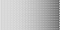Please share what you make with this script. Looking forward to see your output

If you found any part of this unclear or difficult to understand, let me know. If you want more tutorials, show some love and throw me your ideas.

Thanks to Dave Coleman, Alessia Mazzarella, Greta Castellana, Tommaso Zennaro, and Mark Frömberg for proof-reading the tutorial. Thanks to Just van Rossum for showing me unexpected outputs of this script.

Enjoy!

Written by

## Roberto Arista

#### Graphic and type designer. My work is based on the serial production of images and the development of design tools. Lecturer @ ISIA Urbino, t]m 2016 alumni

Welcome to a place where words matter. On Medium, smart voices and original ideas take center stage - with no ads in sight. Watch
Follow all the topics you care about, and we’ll deliver the best stories for you to your homepage and inbox. Explore
Get unlimited access to the best stories on Medium — and support writers while you’re at it. Just \$5/month. Upgrade